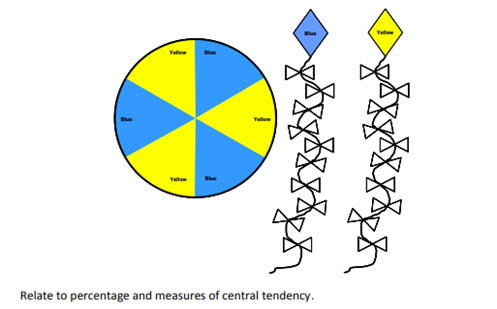# Chances are!

Students calculate the sum of probabilities for a chance experiment and compare frequency predictions with actual data.

Year level(s) Year 5, Year 6
Audience Teacher
Purpose Teaching resource

## Curriculum alignment

Curriculum connections Numeracy
Strand and focus Probability
Topics Chance and probability
AC: Mathematics (V9.0) content descriptions
AC9M5P01
List the possible outcomes of chance experiments involving equally likely outcomes and compare to those which are not equally likely

AC9M6P01

Recognise that probabilities lie on numerical scales of 0 - 1 or 0% - 100% and use estimation to assign probabilities that events occur in a given context, using common fractions, percentages and decimals

Numeracy progression Understanding chance (P3, P4)
Proportional thinking (P1)